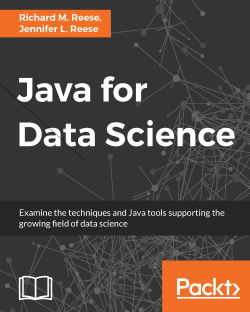# Java for Data Science

By Richard M. Reese , Jennifer L. Reese
• \$41.99 eBook
• \$51.99 Print + eBook
• \$12.99 eBook + Subscription
What do you get with a Packt Subscription?

• Constantly updated with 100+ new titles each month
• Breadth and depth in over 1,000+ technologies

Data science is concerned with extracting knowledge and insights from a wide variety of data sources to analyse patterns or predict future behaviour. It draws from a wide array of disciplines including statistics, computer science, mathematics, machine learning, and data mining. In this book, we cover the important data science concepts and how they are supported by Java, as well as the often statistically challenging techniques, to provide you with an understanding of their purpose and application.

The book starts with an introduction of data science, followed by the basic data science tasks of data collection, data cleaning, data analysis, and data visualization. This is followed by a discussion of statistical techniques and more advanced topics including machine learning, neural networks, and deep learning. The next section examines the major categories of data analysis including text, visual, and audio data, followed by a discussion of resources that support parallel implementation.

The final chapter illustrates an in-depth data science problem and provides a comprehensive, Java-based solution. Due to the nature of the topic, simple examples of techniques are presented early followed by a more detailed treatment later in the book. This permits a more natural introduction to the techniques and concepts presented in the book.

Publication date:
January 2017
Publisher
Packt
Pages
386
ISBN
9781785280115

## Chapter 1. Getting Started with Data Science

Data science is not a single science as much as it is a collection of various scientific disciplines integrated for the purpose of analyzing data. These disciplines include various statistical and mathematical techniques, including:

• Computer science

• Data engineering

• Visualization

• Domain-specific knowledge and approaches

With the advent of cheaper storage technology, more and more data has been collected and stored permitting previously unfeasible processing and analysis of data. With this analysis came the need for various techniques to make sense of the data. These large sets of data, when used to analyze data and identify trends and patterns, become known as big data.

This in turn gave rise to cloud computing and concurrent techniques such as map-reduce, which distributed the analysis process across a large number of processors, taking advantage of the power of parallel processing.

The process of analyzing big data is not simple and evolves to the specialization of developers who were known as data scientists. Drawing upon a myriad of technologies and expertise, they are able to analyze data to solve problems that previously were either not envisioned or were too difficult to solve.

Early big data applications were typified by the emergence of search engines capable of more powerful and accurate searches than their predecessors. For example, AltaVista was an early popular search engine that was eventually superseded by Google. While big data applications were not limited to these search engine functionalities, these applications laid the groundwork for future work in big data.

The term, data science, has been used since 1974 and evolved over time to include statistical analysis of data. The concepts of data mining and data analytics have been associated with data science. Around 2008, the term data scientist appeared and was used to describe a person who performs data analysis. A more in-depth discussion of the history of data science can be found at http://www.forbes.com/sites/gilpress/2013/05/28/a-very-short-history-of-data-science/#3d9ea08369fd.

This book aims to take a broad look at data science using Java and will briefly touch on many topics. It is likely that the reader may find topics of interest and pursue these at greater depth independently. The purpose of this book, however, is simply to introduce the reader to the significant data science topics and to illustrate how they can be addressed using Java.

There are many algorithms used in data science. In this book, we do not attempt to explain how they work except at an introductory level. Rather, we are more interested in explaining how they can be used to solve problems. Specifically, we are interested in knowing how they can be used with Java.

## Problems solved using data science

The various data science techniques that we will illustrate have been used to solve a variety of problems. Many of these techniques are motivated to achieve some economic gain, but they have also been used to solve many pressing social and environmental problems. Problem domains where these techniques have been used include finance, optimizing business processes, understanding customer needs, performing DNA analysis, foiling terrorist plots, and finding relationships between transactions to detect fraud, among many other data-intensive problems.

Data mining is a popular application area for data science. In this activity, large quantities of data are processed and analyzed to glean information about the dataset, to provide meaningful insights, and to develop meaningful conclusions and predictions. It has been used to analyze customer behavior, detecting relationships between what may appear to be unrelated events, and to make predictions about future behavior.

Machine learning is an important aspect of data science. This technique allows the computer to solve various problems without needing to be explicitly programmed. It has been used in self-driving cars, speech recognition, and in web searches. In data mining, the data is extracted and processed. With machine learning, computers use the data to take some sort of action.

## Understanding the data science problem -  solving approach

Data science is concerned with the processing and analysis of large quantities of data to create models that can be used to make predictions or otherwise support a specific goal. This process often involves the building and training of models. The specific approach to solve a problem is dependent on the nature of the problem. However, in general, the following are the high-level tasks that are used in the analysis process:

• Acquiring the data: Before we can process the data, it must be acquired. The data is frequently stored in a variety of formats and will come from a wide range of data sources.

• Cleaning the data: Once the data has been acquired, it often needs to be converted to a different format before it can be used. In addition, the data needs to be processed, or cleaned, so as to remove errors, resolve inconsistencies, and otherwise put it in a form ready for analysis.

• Analyzing the data: This can be performed using a number of techniques including:

• Statistical analysis: This uses a multitude of statistical approaches to provide insight into data. It includes simple techniques and more advanced techniques such as regression analysis.

• AI analysis: These can be grouped as machine learning, neural networks, and deep learning techniques:

• Machine learning approaches are characterized by programs that can learn without being specifically programmed to complete a specific task

• Neural networks are built around models patterned after the neural connection of the brain

• Deep learning attempts to identify higher levels of abstraction within a set of data

• Text analysis: This is a common form of analysis, which works with natural languages to identify features such as the names of people and places, the relationship between parts of text, and the implied meaning of text.

• Data visualization: This is an important analysis tool. By displaying the data in a visual form, a hard-to-understand set of numbers can be more readily understood.

• Video, image, and audio processing and analysis: This is a more specialized form of analysis, which is becoming more common as better analysis techniques are discovered and faster processors become available. This is in contrast to the more common text processing and analysis tasks.

Complementing this set of tasks is the need to develop applications that are efficient. The introduction of machines with multiple processors and GPUs contributes significantly to the end result.

While the exact steps used will vary by application, understanding these basic steps provides the basis for constructing solutions to many data science problems.

### Using Java to support data science

Java and its associated third-party libraries provide a range of support for the development of data science applications. There are numerous core Java capabilities that can be used, such as the basic string processing methods. The introduction of lambda expressions in Java 8 helps enable more powerful and expressive means of building applications. In many of the examples that follow in subsequent chapters, we will show alternative techniques using lambda expressions.

There is ample support provided for the basic data science tasks. These include multiple ways of acquiring data, libraries for cleaning data, and a wide variety of analysis approaches for tasks such as natural language processing and statistical analysis. There are also myriad of libraries supporting neural network types of analysis.

Java can be a very good choice for data science problems. The language provides both object-oriented and functional support for solving problems. There is a large developer community to draw upon and there exist multiple APIs that support data science tasks. These are but a few reasons as to why Java should be used.

The remainder of this chapter will provide an overview of the data science tasks and Java support demonstrated in the book. Each section is only able to present a brief introduction to the topics and the available support. The subsequent chapter will go into considerably more depth regarding these topics.

## Acquiring data for an application

Data acquisition is an important step in the data analysis process. When data is acquired, it is often in a specialized form and its contents may be inconsistent or different from an application's need. There are many sources of data, which are found on the Internet. Several examples will be demonstrated in Chapter 2, Data Acquisition.

Data may be stored in a variety of formats. Popular formats for text data include HTML, Comma Separated Values (CSV), JavaScript Object Notation (JSON), and XML. Image and audio data are stored in a number of formats. However, it is frequently necessary to convert one data format into another format, typically plain text.

For example, JSON (http://www.JSON.org/) is stored using blocks of curly braces containing key-value pairs. In the following example, parts of a YouTube result is shown:

```    {
"etag": etag,
"id": {
"kind": string,
"videoId": string,
"channelId": string,
"playlistId": string
},
...
}
```

Data is acquired using techniques such as processing live streams, downloading compressed files, and through screen scraping, where the information on a web page is extracted. Web crawling is a technique where a program examines a series of web pages, moving from one page to another, acquiring the data that it needs.

With many popular media sites, it is necessary to acquire a user ID and password to access data. A commonly used technique is OAuth, which is an open standard used to authenticate users to many different websites. The technique delegates access to a server resource and works over HTTPS. Several companies use OAuth 2.0, including PayPal, Facebook, Twitter, and Yelp.

## The importance and process of cleaning data

Once the data has been acquired, it will need to be cleaned. Frequently, the data will contain errors, duplicate entries, or be inconsistent. It often needs to be converted to a simpler data type such as text. Data cleaning is often referred to as data wrangling, reshaping, or munging. They are effectively synonyms.

When data is cleaned, there are several tasks that often need to be performed, including checking its validity, accuracy, completeness, consistency, and uniformity. For example, when the data is incomplete, it may be necessary to provide substitute values.

Consider CSV data. It can be handled in one of several ways. We can use simple Java techniques such as the `String` class' `split` method. In the following sequence, a string array, `csvArray`, is assumed to hold comma-delimited data. The `split` method populates a second array, `tokenArray`.

```for(int i=0; i<csvArray.length; i++) {
tokenArray[i] = csvArray[i].split(",");
}
```

More complex data types require APIs to retrieve the data. For example, in Chapter 3, Data Cleaning, we will use the Jackson Project (https://github.com/FasterXML/jackson) to retrieve fields from a JSON file. The example uses a file containing a JSON-formatted presentation of a person, as shown next:

```{
"firstname":"Smith",
"lastname":"Peter",
"phone":8475552222,
}```

The code sequence that follows shows how to extract the values for fields of a person. A parser is created, which uses `getCurrentName` to retrieve a field name. If the name is `firstname`, then the `getText` method returns the value for that field. The other fields are handled in a similar manner.

```try {
JsonFactory jsonfactory = new JsonFactory();
JsonParser parser = jsonfactory.createParser(
new File("Person.json"));
while (parser.nextToken() != JsonToken.END_OBJECT) {
String token = parser.getCurrentName();
if ("firstname".equals(token)) {
parser.nextToken();
String fname = parser.getText();
out.println("firstname : " + fname);
}
...
}
parser.close();
} catch (IOException ex) {
// Handle exceptions
}
```

The output of this example is as follows:

```firstname : Smith
```

Simple data cleaning may involve converting the text to lowercase, replacing certain text with blanks, and removing multiple whitespace characters with a single blank. One way of doing this is shown next, where a combination of the `String` class' `toLowerCase`, `replaceAll`, and `trim` methods are used. Here, a string containing dirty text is processed:

```dirtyText = dirtyText
.toLowerCase()
.replaceAll("[\\d[^\\w\\s]]+", "
.trim();
while(dirtyText.contains("  ")){
dirtyText = dirtyText.replaceAll("  ", " ");
}
```

Stop words are words such as the, and, or but that do not always contribute to the analysis of text. Removing these stop words can often improve the results and speed up the processing.

The LingPipe API can be used to remove stop words. In the next code sequence, a `TokenizerFactory` class instance is used to tokenize text. Tokenization is the process of returning individual words. The `EnglishStopTokenizerFactory` class is a special tokenizer that removes common English stop words.

```text = text.toLowerCase().trim();
TokenizerFactory fact = IndoEuropeanTokenizerFactory.INSTANCE;
fact = new EnglishStopTokenizerFactory(fact);
Tokenizer tok = fact.tokenizer(
text.toCharArray(), 0, text.length());
for(String word : tok){
out.print(word + " ");
}
```

Consider the following text, which was pulled from the book, Moby Dick:

`Call me Ishmael. Some years ago- never mind how long precisely - having little or no money in my purse, and nothing particular to interest me on shore, I thought I would sail about a little and see the watery part of the world.`

The output will be as follows:

```call me ishmael . years ago - never mind how long precisely - having little money my purse , nothing particular interest me shore , i thought i sail little see watery part world .
```

These are just a couple of the data cleaning tasks discussed in Chapter 3, Data Cleaning.

## Visualizing data to enhance understanding

The analysis of data often results in a series of numbers representing the results of the analysis. However, for most people, this way of expressing results is not always intuitive. A better way to understand the results is to create graphs and charts to depict the results and the relationship between the elements of the result.

The human mind is often good at seeing patterns, trends, and outliers in visual representation. The large amount of data present in many data science problems can be analyzed using visualization techniques. Visualization is appropriate for a wide range of audiences ranging from analysts to upper-level management to clientele. In this chapter, we present various visualization techniques and demonstrate how they are supported in Java.

In Chapter 4, Data Visualization, we illustrate how to create different types of graphs, plots, and charts. These examples use JavaFX using a free library called GRAL(http://trac.erichseifert.de/gral/).

Visualization allows users to examine large datasets in ways that provide insights that are not present in the mass of the data. Visualization tools helps us identify potential problems or unexpected data results and develop meaningful interpretations of the data.

For example, outliers, which are values that lie outside of the normal range of values, can be hard to spot from a sea of numbers. Creating a graph based on the data allows users to quickly see outliers. It can also help spot errors quickly and more easily classify data.

For example, the following chart might suggest that the upper two values should be outliers that need to be dealt with: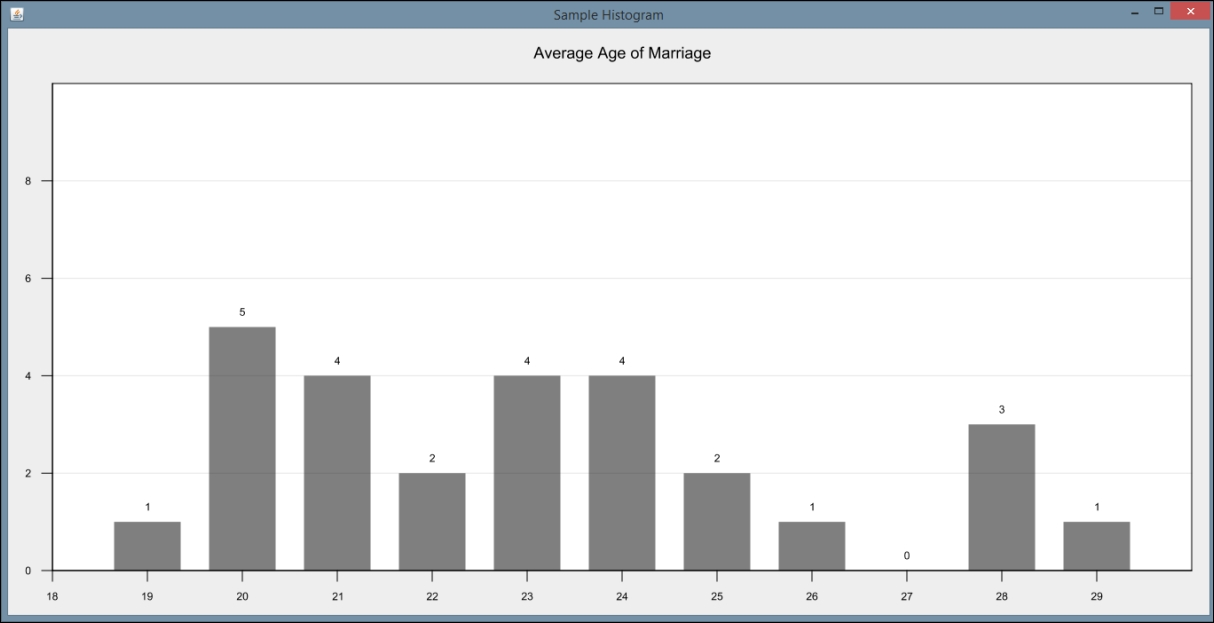## The use of statistical methods in data science

Statistical analysis is the key to many data science tasks. It is used for many types of analysis ranging from the computation of simple mean and medium to complex multiple regression analysis. Chapter 5, Statistical Data Analysis Techniques, introduces this type of analysis and the Java support available.

Statistical analysis is not always an easy task. In addition, advanced statistical techniques often require a particular mindset to fully comprehend, which can be difficult to learn. Fortunately, many techniques are not that difficult to use and various libraries mitigate some of these techniques' inherent complexity.

Regression analysis, in particular, is an important technique for analyzing data. The technique attempts to draw a line that matches a set of data. An equation representing the line is calculated and can be used to predict future behavior. There are several types of regression analysis, including simple and multiple regression. They vary by the number of variables being considered.

The following graph shows the straight line that closely matches a set of data points representing the population of Belgium over several decades: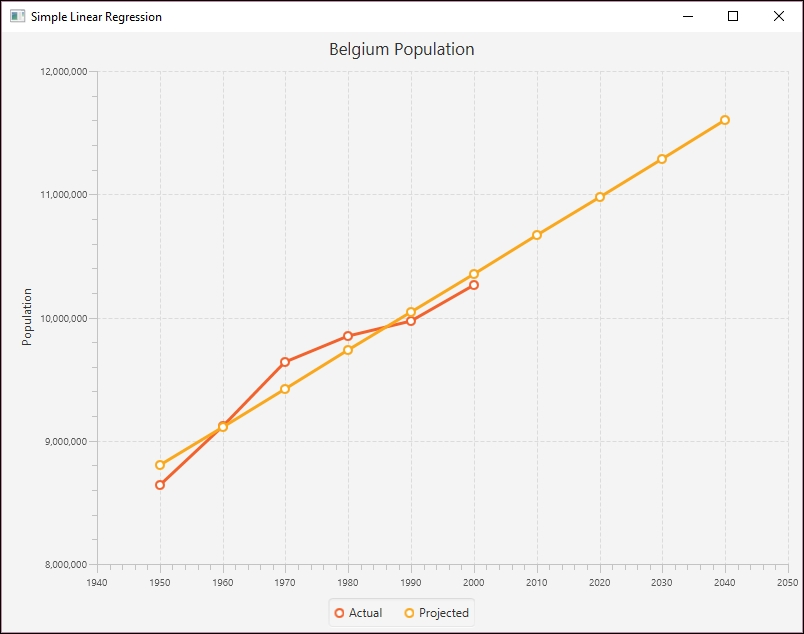Simple statistical techniques, such as mean and standard deviation, can be computed using basic Java. They can also be handled by libraries such as Apache Commons. For example, to calculate the median, we can use the Apache Commons `DescriptiveStatistics` class. This is illustrated next where the median of an array of doubles is calculated. The numbers are added to an instance of this class, as shown here:

```double[] testData = {12.5, 18.3, 11.2, 19.0, 22.1, 14.3, 16.2,
12.5, 17.8, 16.5, 12.5};
DescriptiveStatistics statTest =
new SynchronizedDescriptiveStatistics();
for(double num : testData){
}
```

The `getPercentile` method returns the value stored at the percentile specified in its argument. To find the median, we use the value of `50`.

```out.println("The median is " + statTest.getPercentile(50));

```

Our output is as follows:

```The median is 16.2
```

In Chapter 5, Statistical Data Analysis Techniques, we will demonstrate how to perform regression analysis using the Apache Commons `SimpleRegression` class.

## Machine learning applied to data science

Machine learning has become increasingly important for data science analysis as it has been for a multitude of other fields. A defining characteristic of machine learning is the ability of a model to be trained on a set of representative data and then later used to solve similar problems. There is no need to explicitly program an application to solve the problem. A model is a representation of the real-world object.

For example, customer purchases can be used to train a model. Subsequently, predictions can be made about the types of purchases a customer might subsequently make. This allows an organization to tailor ads and coupons for a customer and potentially providing a better customer experience.

Training can be performed in one of several different approaches:

• Supervised learning: The model is trained with annotated, labeled, data showing corresponding correct results

• Unsupervised learning: The data does not contain results, but the model is expected to find relationships on its own

• Semi-supervised: A small amount of labeled data is combined with a larger amount of unlabeled data

• Reinforcement learning: This is similar to supervised learning, but a reward is provided for good results

There are several approaches that support machine learning. In Chapter 6, Machine Learning, we will illustrate three techniques:

• Decision trees: A tree is constructed using features of the problem as internal nodes and the results as leaves

• Support vector machines: This is used for classification by creating a hyperplane that partitions the dataset and then makes predictions

• Bayesian networks: This is used to depict probabilistic relationships between events

A Support Vector Machine (SVM) is used primarily for classification type problems. The approach creates a hyperplane to categorize data, which can be envisioned as a geometric plane that separates two regions. In a two-dimensional space, it will be a line. In a three-dimensional space, it will be a two-dimensional plane. In Chapter 6, Machine Learning, we will demonstrate how to use the approach using a set of data relating to the propensity of individuals to camp. We will use the Weka class, `SMO`, to demonstrate this type of analysis.

The following figure depicts a hyperplane using a distribution of two types of data points. The lines represent possible hyperplanes that separate these points. The lines clearly separate the data points except for one outlier.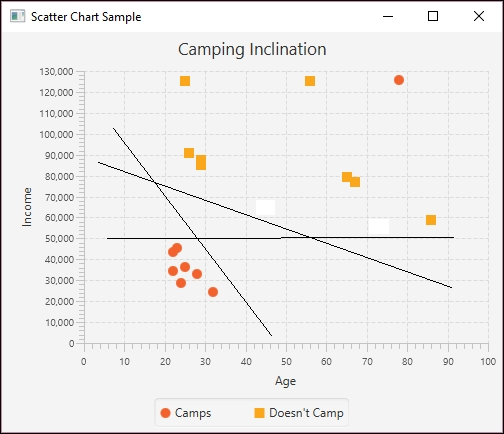Once the model has been trained, the possible hyperplanes are considered and predictions can then be made using similar data.

## Using neural networks in data science

An Artificial Neural Network (ANN), which we will call a neural network, is based on the neuron found in the brain. A neuron is a cell that has dendrites connecting it to input sources and other neurons. Depending on the input source, a weight allocated to a source, the neuron is activated, and then fires a signal down a dendrite to another neuron. A collection of neurons can be trained to respond to a set of input signals.

An artificial neuron is a node that has one or more inputs and a single output. Each input has a weight assigned to it that can change over time. A neural network can learn by feeding an input into a network, invoking an activation function, and comparing the results. This function combines the inputs and creates an output. If outputs of multiple neurons match the expected result, then the network has been trained correctly. If they don't match, then the network is modified.

A neural network can be visualized as shown in the following figure, where Hidden Layer is used to augment the process: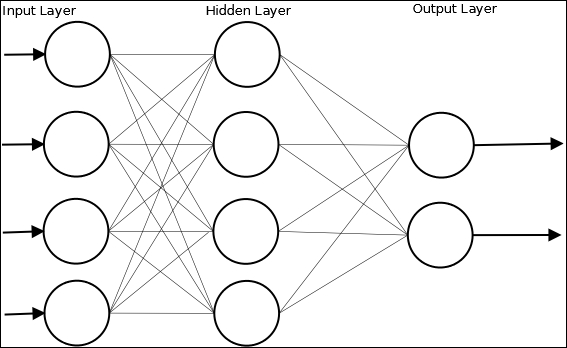In Chapter 7, Neural Networks, we will use the `Weka` class, `MultilayerPerceptron`, to illustrate the creation and use of a Multi Layer Perceptron (MLP) network. As we will explain, this type of network is a feedforward neural network with multiple layers. The network uses supervised learning with backpropagation. The example uses a dataset called `dermatology.arff` that contains 366 instances that are used to diagnose erythemato-squamous diseases. It uses 34 attributes to classify the disease into one of the five different categories.

The dataset is split into a training set and a testing set. Once the data has been read, the MLP instance is created and initialized using the method to configure the attributes of the model, including how quickly the model is to learn and the amount of time spent training the model.

```String trainingFileName = "dermatologyTrainingSet.arff";
String testingFileName = "dermatologyTestingSet.arff";

trainingInstances.setClassIndex(
trainingInstances.numAttributes() - 1);
testingInstances.setClassIndex(
testingInstances.numAttributes() - 1);

MultilayerPerceptron mlp = new MultilayerPerceptron();
mlp.setLearningRate(0.1);
mlp.setMomentum(0.2);
mlp.setTrainingTime(2000);
mlp.setHiddenLayers("3");
mlp.buildClassifier(trainingInstances);
...
} catch (Exception ex) {
// Handle exceptions
}
```

The model is then evaluated using the testing data:

```Evaluation evaluation = new Evaluation(trainingInstances);
evaluation.evaluateModel(mlp, testingInstances);
```

The results can then be displayed:

```System.out.println(evaluation.toSummaryString());
```

The truncated output of this example is shown here where the number of correctly and incorrectly identified diseases are listed:

```Correctly Classified Instances 73 98.6486 %

Incorrectly Classified Instances 1 1.3514 %
```

The various attributes of the model can be tweaked to improve the model. In Chapter 7, Neural Networks, we will discuss this and other techniques in more depth.

## Deep learning approaches

Deep learning networks are often described as neural networks that use multiple intermediate layers. Each layer will train on the outputs of a previous layer potentially identifying features and subfeatures of a dataset. The features refer to those aspects of the data that may be of interest. In Chapter 8, Deep Learning, we will examine these types of networks and how they can support several different data science tasks.

These networks often work with unstructured and unlabeled datasets, which is the vast majority of the data available today. A typical approach is to take the data, identify features, and then use these features and their corresponding layers to reconstruct the original dataset, thus validating the network. The Restricted Boltzmann Machines (RBM) is a good example of the application of this approach.

The deep learning network needs to ensure that the results are accurate and minimizes any error that can creep into the process. This is accomplished by adjusting the internal weights assigned to neurons based on what is known as gradient descent. This represents the slope of the weight changes. The approach modifies the weight so as to minimize the error and also speeds up the learning process.

There are several types of networks that have been classified as a deep learning network. One of these is an autoencoder network. In this network, the layers are symmetrical where the number of input values is the same as the number of output values and the intermediate layers effectively compress the data to a single smaller internal layer. Each layer of the autoencoder is a RBM.

This structure is reflected in the following example, which will extract the numbers found in a set of images containing hand-written numbers. The details of the complete example are not shown here, but notice that 1,000 input and output values are used along with internal layers consisting of RBMs. The size of the layers are specified in the `nOut` and `nIn` methods.

```MultiLayerConfiguration conf = new NeuralNetConfiguration.Builder()
.seed(seed)
.iterations(numberOfIterations)
.optimizationAlgo(
.list()
.layer(0, new RBM.Builder()
.nIn(numberOfRows * numberOfColumns).nOut(1000)
.lossFunction(LossFunctions.LossFunction.RMSE_XENT)
.build())
.layer(1, new RBM.Builder().nIn(1000).nOut(500)
.lossFunction(LossFunctions.LossFunction.RMSE_XENT)
.build())
.layer(2, new RBM.Builder().nIn(500).nOut(250)
.lossFunction(LossFunctions.LossFunction.RMSE_XENT)
.build())
.layer(3, new RBM.Builder().nIn(250).nOut(100)
.lossFunction(LossFunctions.LossFunction.RMSE_XENT)
.build())
.layer(4, new RBM.Builder().nIn(100).nOut(30)
.lossFunction(LossFunctions.LossFunction.RMSE_XENT)
.build()) //encoding stops
.layer(5, new RBM.Builder().nIn(30).nOut(100)
.lossFunction(LossFunctions.LossFunction.RMSE_XENT)
.build()) //decoding starts
.layer(6, new RBM.Builder().nIn(100).nOut(250)
.lossFunction(LossFunctions.LossFunction.RMSE_XENT)
.build())
.layer(7, new RBM.Builder().nIn(250).nOut(500)
.lossFunction(LossFunctions.LossFunction.RMSE_XENT)
.build())
.layer(8, new RBM.Builder().nIn(500).nOut(1000)
.lossFunction(LossFunctions.LossFunction.RMSE_XENT)
.build())
.layer(9, new OutputLayer.Builder(
LossFunctions.LossFunction.RMSE_XENT).nIn(1000)
.nOut(numberOfRows * numberOfColumns).build())
.pretrain(true).backprop(true)
.build();
```

Once the model has been trained, it can be used for predictive and searching tasks. With a search, the compressed middle layer can be used to match other compressed images that need to be classified.

## Performing text analysis

The field of Natural Language Processing (NLP) is used for many different tasks including text searching, language translation, sentiment analysis, speech recognition, and classification to mention a few. Processing text is difficult due to a number of reasons, including the inherent ambiguity of natural languages.

There are several different types of processing that can be performed such as:

• Identifying Stop words: These are words that are common and may not be necessary for processing

• Name Entity Recognition (NER): This is the process of identifying elements of text such as people's names, location, or things

• Parts of Speech (POS): This identifies the grammatical parts of a sentence such as noun, verb, adjective, and so on

• Relationships: Here we are concerned with identifying how parts of text are related to each other, such as the subject and object of a sentence

As with most data science problems, it is important to preprocess and clean text. In Chapter 9, Text Analysis, we examine the support Java provides for this area of data science.

For example, we will use Apache's OpenNLP (https://opennlp.apache.org/) library to find the parts of speech. This is just one of the several NLP APIs that we could have used including LingPipe (http://alias-i.com/lingpipe/), Apache UIMA (https://uima.apache.org/), and Standford NLP (http://nlp.stanford.edu/). We chose OpenNLP because it is easy to use for this example.

In the following example, a model used to identify POS elements is found in the `en-pos-maxent.bin` file. An array of words is initialized and the POS model is created:

```try (InputStream input = new FileInputStream(
new File("en-pos-maxent.bin"));) {
String sentence = "Let's parse this sentence.";
...
String[] words;
...
list.toArray(words);
POSModel posModel = new POSModel(input);
...
} catch (IOException ex) {
// Handle exceptions
}
```

The `tag` method is passed an array of `words` and returns an array of tags. The words and tags are then displayed.

```String[] posTags = posTagger.tag(words);
for(int i=0; i<posTags.length; i++) {
out.println(words[i] + " - " + posTags[i]);
}
```

The output for this example is as follows:

```Let's - NNP

parse - NN

this - DT

sentence. - NN
```

The abbreviations `NNP` and `DT` stand for a singular proper noun and determiner respectively. We examine several other NLP techniques in Chapter 9, Text Analysis.

## Visual and audio analysis

In Chapter 10, Visual and Audio Analysis, we demonstrate several Java techniques for processing sounds and images. We begin by demonstrating techniques for sound processing, including speech recognition and text-to-speech APIs. Specifically, we will use the FreeTTS (http://freetts.sourceforge.net/docs/index.php) API to convert text to speech. We also include a demonstration of the CMU Sphinx toolkit for speech recognition.

The Java Speech API (JSAPI) (http://www.oracle.com/technetwork/java/index-140170.html) supports speech technology. This API, created by third-party vendors, supports speech recognition and speech synthesizers. FreeTTS and Festival (http://www.cstr.ed.ac.uk/projects/festival/) are examples of vendors supporting JSAPI.

In the second part of the chapter, we examine image processing techniques such as facial recognition. This demonstration involves identifying faces within an image and is easy to accomplish using OpenCV (http://opencv.org/).

Also, in Chapter 10, Visual and Audio Analysis, we demonstrate how to extract text from images, a process known as OCR. A common data science problem involves extracting and analyzing text embedded in an image. For example, the information contained in license plate, road signs, and directions can be significant.

In the following example, explained in more detail in Chapter 11Mathematical and Parallel Techniques for Data Analysis accomplishes OCR using Tess4j (http://tess4j.sourceforge.net/) a Java JNA wrapper for Tesseract OCR API. We perform OCR on an image captured from the Wikipedia article on OCR (https://en.wikipedia.org/wiki/Optical_character_recognition#Applications), shown here: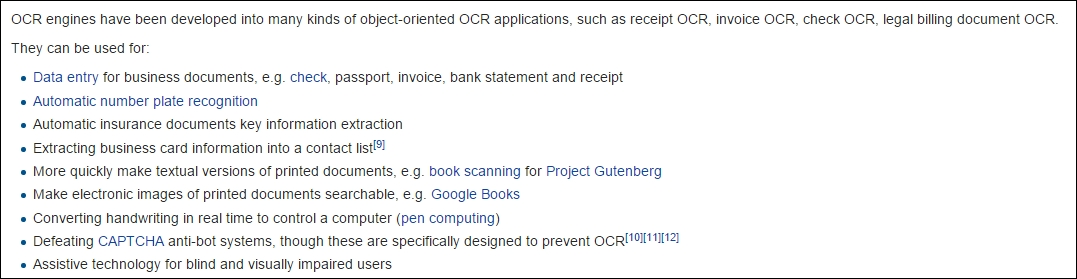The `ITesseract` interface provides numerous OCR methods. The `doOCR` method takes a file and returns a string containing the words found in the file as shown here:

```ITesseract instance = new Tesseract();
try {
String result = instance.doOCR(new File("OCRExample.png"));
System.out.println(result);
} catch (TesseractException e) {
System.err.println(e.getMessage());
}
```

A part of the output is shown next:

```OCR engines nave been developed into many lunds oiobiectorlented OCR applicatlons, sucn as reoeipt OCR, involoe OCR, check OCR, legal billing document OCR

They can be used ior

- Data entry ior business documents, e g check, passport, involoe, bank statement and receipt

- Automatic number plate recognnlon
```

As you can see, there are numerous errors in this example that need to be addressed. We build upon this example in Chapter 11, Mathematical and Parallel Techniques for Data Analysis, with a discussion of enhancements and considerations to ensure the OCR process is as effective as possible.

We will conclude the chapter with a discussion of NeurophStudio, a neural network Java-based editor, to classify images and perform image recognition. We train a neural network to recognize and classify faces in this section.

## Improving application performance using parallel techniques

In Chapter 11, Mathematical and Parallel Techniques for Data Analysis, we consider some of the parallel techniques available for data science applications. Concurrent execution of a program can significantly improve performance. In relation to data science, these techniques range from low-level mathematical calculations to higher-level API-specific options.

This chapter includes a discussion of basic performance enhancement considerations. Algorithms and application architecture matter as much as enhanced code, and this should be considered when attempting to integrate parallel techniques. If an application does not behave in the expected or desired manner, any gains from parallel optimizing are irrelevant.

Matrix operations are essential to many data applications and supporting APIs. We will include a discussion in this chapter about matrix multiplication and how it is handled using a variety of approaches. Even though these operations are often hidden within the API, it can be useful to understand how they are supported.

One approach we demonstrate utilizes the Apache Commons Math API (http://commons.apache.org/proper/commons-math/). This API supports a large number of mathematical and statistical operations, including matrix multiplication. The following example illustrates how to perform matrix multiplication.

We first declare and initialize matrices `A` and `B`:

```double[][] A = {
{0.1950, 0.0311},
{0.3588, 0.2203},
{0.1716, 0.5931},
{0.2105, 0.3242}};

double[][] B = {
{0.0502, 0.9823, 0.9472},
{0.5732, 0.2694, 0.916}};
```

Apache Commons uses the `RealMatrix` class to store a matrix. Next, we use the `Array2DRowRealMatrix` constructor to create the corresponding matrices for `A` and `B`:

```RealMatrix aRealMatrix = new Array2DRowRealMatrix(A);
RealMatrix bRealMatrix = new Array2DRowRealMatrix(B);
```

We perform multiplication simply using the `multiply` method:

```RealMatrix cRealMatrix = aRealMatrix.multiply(bRealMatrix);
```

Finally, we use a `for` loop to display the results:

```for (int i = 0; i < cRealMatrix.getRowDimension(); i++) {
System.out.println(cRealMatrix.getRowVector(i));
}
```

The output is as follows:

```{0.02761552; 0.19992684; 0.2131916}
{0.14428772; 0.41179806; 0.54165016}
{0.34857924; 0.32834382; 0.70581912}
{0.19639854; 0.29411363; 0.4963528}
```

Another approach to concurrent processing involves the use of Java threads. Threads are used by APIs such as Aparapi when multiple CPUs or GPUs are not available.

Data science applications often take advantage of the map-reduce algorithm. We will demonstrate parallel processing by using Apache's Hadoop to perform map-reduce. Designed specifically for large datasets, Hadoop reduces processing time for large scale data science projects. We demonstrate a technique for calculating the average value of a large dataset.

We also include examples of APIs that support multiple processors, including CUDA and OpenCL. CUDA is supported using Java bindings for CUDA (JCuda) (http://jcuda.org/). We also discuss OpenCL and its Java support. The Aparapi API provides high-level support for using multiple CPUs or GPUs and we include a demonstration of Aparapi in support of matrix multiplication.

## Assembling the pieces

In the final chapter of this book, we will tie together many of the techniques explored in the previous chapters. We will create a simple console-based application for acquiring data from Twitter and performing various types of data manipulation and analysis. Our goal in this chapter is to demonstrate a simple project exploring a variety of data science concepts and provide insights and considerations for future projects.

Specifically, the application developed in the final chapter performs several high-level tasks, including data acquisition, data cleaning, sentiment analysis, and basic statistical collection. We demonstrate these techniques using Java 8 Streams and focus on Java 8 approaches whenever possible.

## Summary

Data science is a broad, diverse field of study and it would be impossible to explore exhaustively within this book. We hope to provide a solid understanding of important data science concepts and equip the reader for further study. In particular, this book will provide concrete examples of different techniques for all stages of data science related inquiries. This ranges from data acquisition and cleaning to detailed statistical analysis.

So let's start with a discussion of data acquisition and how Java supports it as illustrated in the next chapter.